# Heat and thermodynamics MCQ

In this page we have Multiple Choice questions on heat and thermodynamics for JEE Main and Advanced . Hope you like them and do not forget to like , social share and comment at the end of the page.

## Multiple choice questions with one or more answers

Question 1
It is known that curves A, B, C are Isobaric, Isothermal, Adiabatic process then when one is correct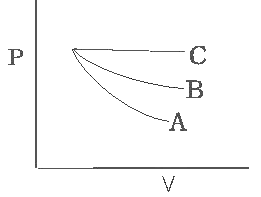(a) A - Adiabatic, B - Isothermal,, C - Isobaric
(b) A- Isothermal,, B - Adiabatic, C - Isobaric
(c) A - Isobaric, B - Isothermal C - Adiabatic
(d) None of these

Question 2
Which of the following graph correctly represent the variation
$\delta = \frac {dV }{V dT}$
for an ideal gas at constant pressure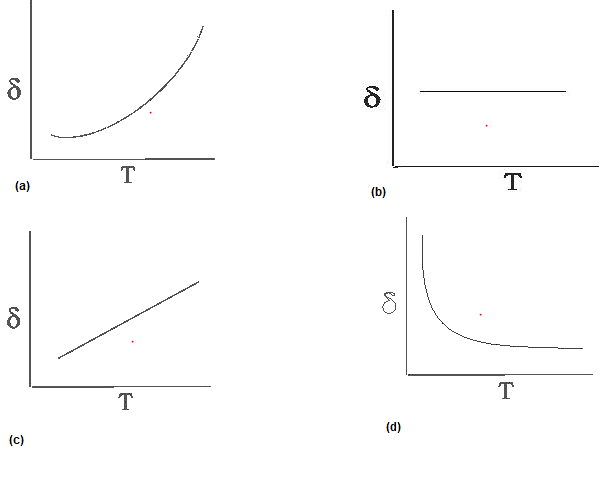Question 3
An ideal gas taken round the cycle ABCA as shown in PV diagram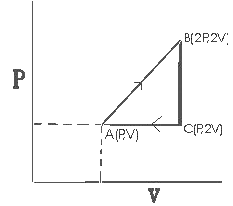The work done during the cycle,
a. $PV$
b. $\frac {PV}{2}$
c. $2PV$
d. $\frac {PV}{3}$

Question 4
Consider the following statements

(Assertion) The internal energy of an ideal gas does not change during an Isothermal process
(Reason) The decrease in the volume of the gas is compensated by a corresponding increase in pressure when its temperature is constant in accordance with Boyle law

a. Both A & R are true and R is correct Explanation of A
b. Both A & R are true and R is not correct Explanation of A
c. A is true R is false
d. A is false but R is true

Question 5
A thermally insulated vessel containing an gas when molar mass is M and Ratio of specific heat Cp / Cv = γ move with a velocity V.The gas temperature increase by ΔT due to sudden stoppage of vessel. Find the value of V in terms of ΔT, M, γ
a. $V = [\frac {2R \Delta T} {M (\gamma-1)}]^{1/2}$
b. $V = [\frac {2R \Delta T}{ M (\gamma + 1)}]^{1/2}$
c. $V = [\frac {2R \Delta T}{ M \sqrt {(\gamma-1)}}]^{1/2}$
d. $V = [\frac {2R \Delta T}{ M \sqrt {(\gamma+1)}}]^{1/2}$

Question 6
Match the column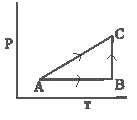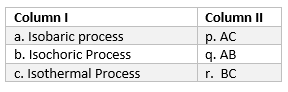a. a-> q, b -> p, c-> r
b. a-> p, b -> q, c-> r
c. a-> r, b -> q, c-> p
d. a-> p, b -> r, c-> q

Question 7
An ideal gas is taken through a cyclic thermodynamics process through four steps.
The amount of heat involved in the steps are Q1 = 5960 J,Q2 = - 5600 J,Q3= -3000 J,Q4 = -3600 J
respectively. The corresponding quantities of Internal energy changes are ΔU1 = 3.760 J ,ΔU2 = - 4800 J,ΔU3 = -1800 J,ΔU4 = ?
find the value ΔU4 & net work done
a. 2930 J, 960 J
b. 2830 J, 900 J
C. 2930 J, -960 J
d. -2930 J, 960 J

Question 8
An ideal gas who ratio of specific heat Cp / Cv = γ is Expanded according to the law P = av2
when a is constant. The ratio of final volume to Initial value is n .find the ΔU.Initial volume is V0
a. aV03 (n3-1) / γ-1
b. aV02 (n2 - 1) / γ-1
c. aV03 (n3 + 1) /γ+1
d. aV02 (n2 + 1) /γ-1

Question 9
During an adiabatic process the square of the pressure of a gas is proportional to the fifth power of its absolute temperature. The ratio of specific heat Cp / Cv for that gas is
a. 3/5
b. 4/3
c. 5/3
d. 3/2

Question 10
A vessel contains 4 mole of O2(relative molar mass 32) at temperature T. The pressure is P. An identical vessel contains 1 mole of nitrogen of temperature 2T. find the pressure the
a. P/2
b. 2P
c. 8P
d. P

Question 11
What is the molar specific heat of a isothermal & adiabatic process respectively
a. ∞ , 0
b. 0, ∞
c. 0, 0
d. none of these

Question 12
An ideal gas has molar specific heat at constant volume = Cv. find the molar heat capacity of this gas as a function of volume V at the gas goes through process.
$T = T_0(e^{aV} + 1)$
a. $C_v + (\frac {R}{aV}) + (\frac {R}{aVe^{aV}})$
b. $C_v + (\frac {R}{aV})$
c. $C_v + (\frac {R^2}{aV})$
d. $C_v - (\frac {R^2}{aV})$

## Linked Comprehension type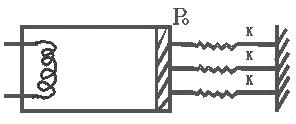1 mole of a diatomic idea gas is enclosed in a adiabatic cylinder filled with a smooth light adiabatic Piston. The Piston is connected to three spring of spring constant K as shown in figure. The area of cross-section of Cylinder is A Initially spring is in its natural length and atmosphere pressure is P0

Question 13
find the pressure of the gas
a. $P_0$
b. $2P_0$
c. $\frac {P_0}{2}$
d. $4P_0$

Question 14
If the heat is supplied to the gas and piston move by distance L due to that then what is the work done by the gas
a. $P_0AL+ \frac {3}{2}KL^2$
b. $P_0AL$
c. $\frac {3}{2}KL^2$
d. $P_0AL- \frac {3}{2}KL^2$

Question 15
if Temp changes by ΔT due to heat transfer and γ=5/2 then Find out the internal energy change
a. $R \Delta T$
b. $\frac {2}{3}R \Delta T$
c.$\frac {5}{2} R \Delta T$
d. $\frac {1}{2} R \Delta T$

Question 16
Find the total heat supply
a. $R \Delta T +\frac {3}{2}KL^2$
b. $P_0 AL+\frac {3}{2}KL^2 + \frac {2}{3} R \Delta T$
c. $R \Delta T -\frac {3}{2}KL^2$
d. $R \Delta T + P_0AL$

## Multiple choice questions with one or more answers

Question 17
One mole of ideal gas having adiabatic coefficient γ1 is mixed with 1 one mole of an ideal gas have adiabatic coefficient γ2
Find the γ of the mixture
a.$\frac {(2 \gamma _1 \gamma _2 - \gamma _1 - \gamma _2 ) }{( \gamma _1 + \gamma _2 - 2)}$
b.$\frac {(2 \gamma _1 \gamma _2 - \gamma _1 - \gamma _2 ) }{( \gamma _1 + \gamma _2 + 2)}$
c.$\frac {(2 \gamma _1 \gamma _2 + \gamma _1 - \gamma _2 ) }{( \gamma _1 + \gamma _2 - 2)}$
d. None of these

Question 18
We have a process defined as $PV^n = constant$
and we have an adiabatic process defined by $PV^{\gamma} = Constant$
and so thermal process defined as $PV = Constant$
find the Ratio of Bulk modules of Poly-tropic, adiabatic, isothermal process
a. $n: \gamma : 1$
b. $1 : n : \gamma$
c. $n^2 : \gamma ^2 : 1$
d. $1 : n^2 : \gamma ^2$

Question 19
Find the wok done in the cyclic process as shown in figure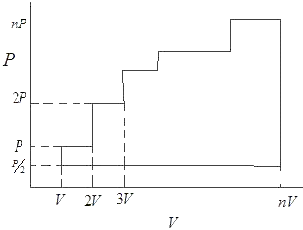a. $\frac {n^2PV}{2}$
b.$\frac {n^2PV}{8}$
c. $\frac {n^2PV}{16}$
d. none of these

Question 20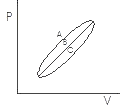Let Q1, Q2 , Q2 heat given to the system in processes A, B, C respectively
consider two statements

a, Q1 > Q2 > Q3
b, WA > WB > WC

1, Both A & B are Correct
2, Both A & B are wrong
3. A is Correct Only
4. B is Correct Only.

Question 21
Match the Column
Column A
a. Isothermal process
c. Isobaric process
d. Isochoric process
Column B
x. ΔU = ΔQ
y. ΔU = ΔQ - ΔW
z. ΔU = -ΔW
w. ΔQ = ΔW

a, a -> x, b-> y, c->z, d->w
b, a->w, b->z, c->x, d->y
c, a->y, b->x, c->z, d->w
d, a->z,b->w,c->y,d->x

Question 22
The ratio of adiabatic bulk modulus and isothermal bulk modulus of a gas ($\gamma = \frac {C_p}{C_v}$) is
a. $\frac {\gamma -1}{\gamma}$
b. 1

c. $\gamma$
d. $\frac {\gamma}{\gamma -1 }$

Question 23
Two boxes A and B containing different ideal gases are placed on table
Box A contain one mole of gas m where (Cv=5R/2) at Temperature T0
Box B contains one mole of gas n where (Cv=3R/2) at temperature (7/3) T0
The boxes are then put into thermal contact with each other and heat flows between until the gases reach a common final temperature Tf
Which of the following relation is correct?
a. 2Tf-3T0=0
b. 2Tf-7T0=0
c. 2Tf-5T0=0
d. Tf-3T0=0

Question 24
Which one of the following statement is true about a gas undergoing isothermal change
a. The temperature of the gas is constant
b. The pressure of the gas  remains constant
c. the volume of the gas remains constant
d. The gas is completely insulated from the surrounding’s

Question 25
Three copper blocks of masses $M_1$, $M_2$ and $M_3$ kg respectively are brought into thermal contact till they reach equilibrium. Before contact, they were at $T_1$, $T_2$, $T_3$ ($T_1 > T_2 > T_3$ ). Assuming there is no heat loss to the surroundings, the equilibrium temperature T is (s is specific heat of copper)
a. $\frac {T_1 + T_2 + T_3}{3}$
b. $\frac {M_1T_1 + M_2T_2 + M_3T_3}{M_1 + M_2 + M+3}$
c. $\frac {M_1T_1 + M_2T_2 + M_3T_3}{3(M_1 + M_2 + M+3)}$
d. $\frac {M_1T_1s + M_2T_2s + M_3T_3s}{3(M_1 + M_2 + M+3)}$

Question 26
One mole of an ideal gas goes through the cyclic process ABCA. Pressure at State A = $P_0$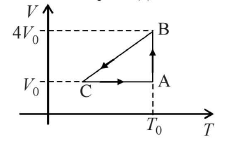Which of the following is correct
a. Pressure at C is $\frac {P_0}{4}$
b. temperature at C is $\frac {T_0}{4}$
c. $W_{AB}=P_0V_0 ln 4$
d. $U_A =$U_B\$

Question 27
The figure shows the P-V plot of an ideal gas taken through a cycle ABCDA. The part ABC is a semi-circle and CDA is half of an ellipse. Then -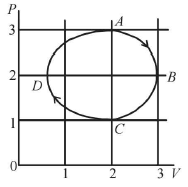a. the process during the path A ? B is isothermal
b. heat flows out of the gas during the path B ? C ? D
c. work done during the path A ? B ? C is zero
d. positive work is done by the gas in the cycle ABCDA